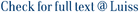Two wavelet based estimators are considered in this paper for the two parameters that characterize long range dependence processes. The first one is linear and is based on the statistical properties of the coefficients of a discrete wavelet transform of long range dependence processes. The estimator consists in measuring the slope (related to the long memory parameter) and the intercept (related to the variance of the process) of a linear regression after a discrete wavelet transform is performed (Veitch and Abry, 1999). In this paper its properties are reviewed, and analytic evidence is produced that the linear estimator is applicable only when the second parameter is unknown. To overcome this limitation a non linear wavelet based estimator – that takes into account that the intercept depends on the long memory parameter – is proposed here for the cases in which the second parameter is known or the only parameter of interest is the long memory parameter. Under the same hypothesis assumed for the linear estimator, the non linear estimator is shown to be asymptotically more efficient for the long memory parameter. Numerical simulations show that, even for small data sets, the bias is very small and the variance close to optimal. An application to ATM based Internet traffic is presented.

A non linear wavelet based estimator for long memory processes / De Giovanni, Livia; Maurizio, Naldi. - In: STATISTICAL METHODS & APPLICATIONS. - ISSN 1618-2510. - 13:1(2004), pp. 27-41.

### A non linear wavelet based estimator for long memory processes

#### Abstract

Two wavelet based estimators are considered in this paper for the two parameters that characterize long range dependence processes. The first one is linear and is based on the statistical properties of the coefficients of a discrete wavelet transform of long range dependence processes. The estimator consists in measuring the slope (related to the long memory parameter) and the intercept (related to the variance of the process) of a linear regression after a discrete wavelet transform is performed (Veitch and Abry, 1999). In this paper its properties are reviewed, and analytic evidence is produced that the linear estimator is applicable only when the second parameter is unknown. To overcome this limitation a non linear wavelet based estimator – that takes into account that the intercept depends on the long memory parameter – is proposed here for the cases in which the second parameter is known or the only parameter of interest is the long memory parameter. Under the same hypothesis assumed for the linear estimator, the non linear estimator is shown to be asymptotically more efficient for the long memory parameter. Numerical simulations show that, even for small data sets, the bias is very small and the variance close to optimal. An application to ATM based Internet traffic is presented.
##### Scheda breve Scheda completa Scheda completa (DC)2004
Wavelet transform; Self similar processes; Long range dependence; Statistical inference; Telecommunication networks
A non linear wavelet based estimator for long memory processes / De Giovanni, Livia; Maurizio, Naldi. - In: STATISTICAL METHODS & APPLICATIONS. - ISSN 1618-2510. - 13:1(2004), pp. 27-41.
File in questo prodotto:
File
5 DeGiovanniNaldi-SMA.pdf

Solo gestori archivio

Tipologia: Documento in Post-print
Licenza: DRM (Digital rights management) non definiti
Dimensione 147.52 kB
abstractSMA2004.pdf

Solo gestori archivio

Tipologia: Abstract
Licenza: DRM (Digital rights management) non definiti
Dimensione 65.14 kB
Utilizza questo identificativo per citare o creare un link a questo documento: `https://hdl.handle.net/11385/6035`
•3
•ND